###Author Topic: Polar Plane?  (Read 6254 times)

0 Members and 1 Guest are viewing this topic.

####imPersonator##### Polar Plane?
« on: July 22, 2010, 09:37:03 am »
I've been trying to get something of a polar plane in Axe using sines and cosines, but am not having any luck.  Is there a good way to do this?

####calcdude84se

• Needs Motivation
• LV11 Super Veteran (Next: 3000)
••• Posts: 2272
• Rating: +78/-13
• Wondering where their free time went...##### Re: Polar Plane?
« Reply #1 on: July 22, 2010, 09:38:58 am »
Meanwhile, I'll bump the feature request for having sin( and cos( support 8.8 FP arguments.
"People think computers will keep them from making mistakes. They're wrong. With computers you make mistakes faster."
I'll put it online when it does something.

####quasi_Phthalo##### Re: Polar Plane?
« Reply #2 on: July 22, 2010, 11:28:56 am »
is this like a plane in 3D, but you can only describe points on it using two angles and a radius?

####imPersonator##### Re: Polar Plane?
« Reply #3 on: July 22, 2010, 11:47:48 am »
I only want this for 2D, so just one angle and a radius.  Points are plotted on a polar plane by the distance from a point and the angle,so by changing the angle but not the distance, you could draw a circle (this is not what I want to use it for.  With a polar plane, it is very easy to make curves and do rotations and such because points are based on angle).  The formula (in degree mode, not radian) is x=distance*cos(angle) , y=distance*sin(angle)

####Deep Toaster

• So much to do, so much time, so little motivation
• LV13 Extreme Addict (Next: 9001)
••• Posts: 8217
• Rating: +758/-15##### Re: Polar Plane?
« Reply #4 on: July 27, 2010, 10:37:03 am »
Are you trying to get parametric equations with polar coordinates (i.e., using r and θ to find x and y)? If so, I tried to make something useful: a few quick programs drawing with them.

The first one (prgmA) is just a simple circle, and the second (prgmB) is one of those parametric roses that they always have to put in textbooks. The third (prgmC) and fourth (prgmD) make several drawings simultaneously to create more complex things (like a yin-yang symbol and calcdude84se's avatar (reversed to save space (and time))).

So far, I've found it's easiest to create a For( loop for θ (to simulate a polar graph), then initiate r (if it's not static; so this only applies to the second program) according to that (again like a polar graph), then draw the pixels individually using the x- and y-equations you gave, but multiplying first by the radius, whatever it is, then dividing by 128 (since the result of sin( and cos( in the Axe language are between -127 and 127) with the // command (to save the sign).

Hope this helpsEDIT: Don't know what went wrong for you, but did you remember the domain and range for Axe's sin( and cos( commands?

EDIT2: If you noticed that the circles are kinda squarish, that's because of all the rounding (all integers, remember).
« Last Edit: July 27, 2010, 10:40:19 am by Deep Thought »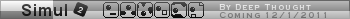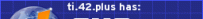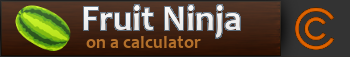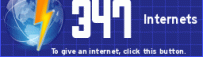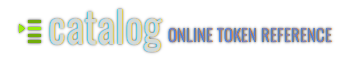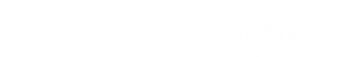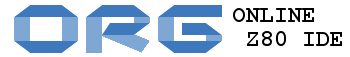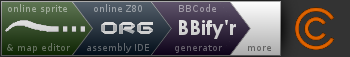####imPersonator##### Re: Polar Plane?
« Reply #5 on: July 27, 2010, 10:53:06 am »
Thanks, it worked, but I'm confused.  Why are there 255 "degrees" (or whatever they are) in a circle instead of 360?  Is it so the sine and cosine fit in a byte?

#### SirCmpwn

• Guest##### Re: Polar Plane?
« Reply #6 on: July 27, 2010, 10:55:21 am »
Well, in Axe, sine and cosine are really sine and cosine * 255.

####Deep Toaster

• So much to do, so much time, so little motivation
• LV13 Extreme Addict (Next: 9001)
••• Posts: 8217
• Rating: +758/-15##### Re: Polar Plane?
« Reply #7 on: July 28, 2010, 07:11:13 am »
Thanks, it worked, but I'm confused.  Why are there 255 "degrees" (or whatever they are) in a circle instead of 360?  Is it so the sine and cosine fit in a byte?

One period (according to the commands list) is 255, so yeah, in a way there are now 256 degrees in a circle (since 256 is the same as 0)It's probably easier to use, since with a period of 255, if you're using 2-byte integers, the high byte doesn't matter.

The answer is multiplied by 127 (again, it fits neatly in a byte, and it works since numbers in Axe are all integers).